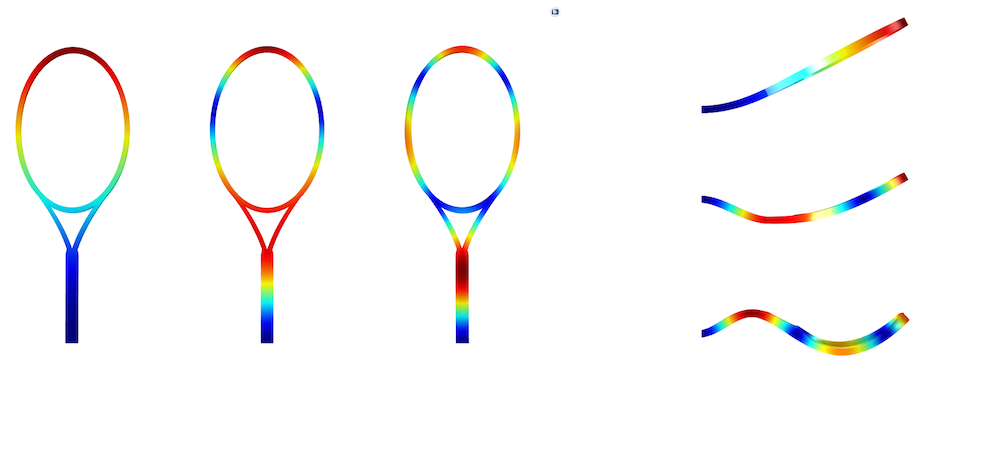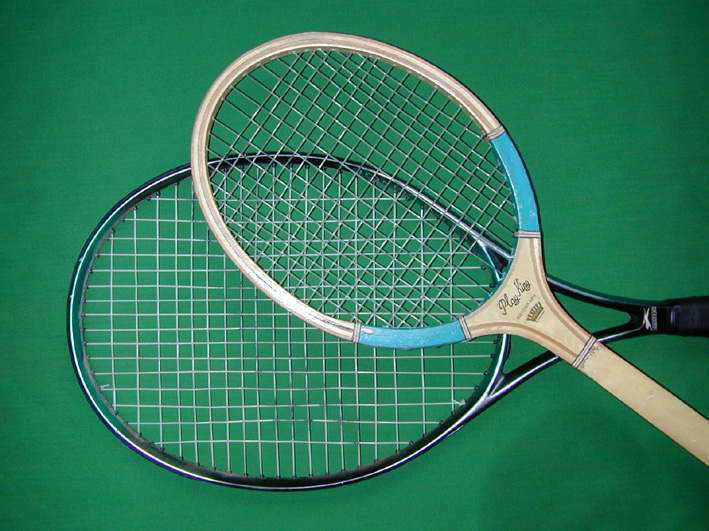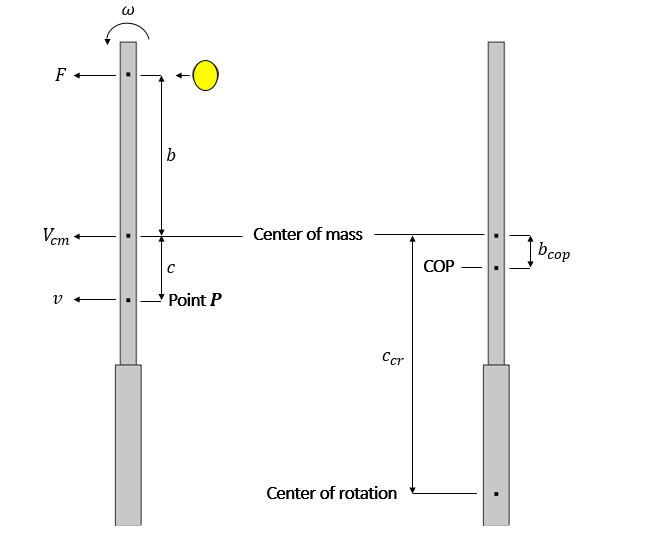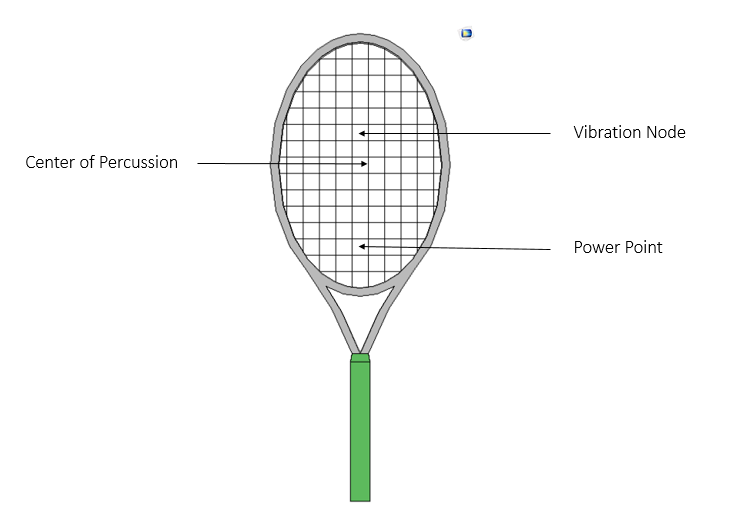# 网球拍“甜区”中的物理学

2015年 8月 27日

### 网球拍的振动节点### 网球拍的撞击中心F=M \frac{d V_{cm}}{dt}

Fb = I\frac{d\omega}{dt}

\frac{dv}{dt}=(\frac{1}{M}-\frac{cb}{I})F

b_{cop}=\frac{I}{c_{cr}M}

### 哑点

\frac{1}{2}mV_0^2 = \frac{1}{2}mV_1^2+\frac{1}{2}mV_2^2
mV_0=mV_1+mV_2

V_1=0 \ \text{and} \ V_2=V_0

### 盘点-局点-破发点